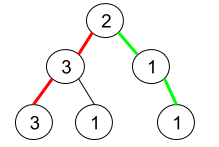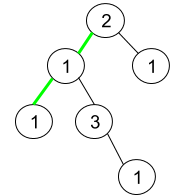# 1457. Pseudo-Palindromic Paths in a Binary Tree | LeetCode Solution

## 1457. Pseudo-Palindromic Paths in a Binary Tree

Given a binary tree where node values are digits from 1 to 9. A path in the binary tree is said to be pseudo-palindromic if at least one permutation of the node values in the path is a palindrome.

Return the number of pseudo-palindromic paths going from the root node to leaf nodes.

Example 1:```Input: root = [2,3,1,3,1,null,1]
Output: 2
Explanation: The figure above represents the given binary tree. There are three paths going from the root node to leaf nodes: the red path [2,3,3], the green path [2,1,1], and the path [2,3,1]. Among these paths only red path and green path are pseudo-palindromic paths since the red path [2,3,3] can be rearranged in [3,2,3] (palindrome) and the green path [2,1,1] can be rearranged in [1,2,1] (palindrome).
```

Example 2:```Input: root = [2,1,1,1,3,null,null,null,null,null,1]
Output: 1
Explanation: The figure above represents the given binary tree. There are three paths going from the root node to leaf nodes: the green path [2,1,1], the path [2,1,3,1], and the path [2,1]. Among these paths only the green path is pseudo-palindromic since [2,1,1] can be rearranged in [1,2,1] (palindrome).
```

Example 3:

```Input: root = 
Output: 1
```

Constraints:

• The number of nodes in the tree is in the range `[1, 105]`.
• `1 <= Node.val <= 9`